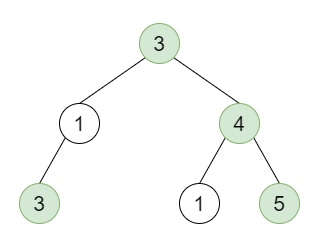# Count Good Nodes in Binary Tree in C++

Suppose we have a binary tree, a node X in the tree is named good when in the path from root to X there are no nodes whose value is greater than X. Here we have to find the number of good nodes in the binary tree.

So, if the input is like,then the output will be 4, the colored nodes are good node.

To solve this, we will follow these steps −

• Define a function dfs(), this will take node, val,

• if node is null, then −

• return

• ret := ret + (1 when val <= val of node, otherwise 0)

• dfs(left of node, maximum of val and val of node)

• dfs(right of node, maximum of val and val of node)

• From the main method do the following −

• ret := 0

• dfs(root, -inf)

• return ret

## Example

Let us see the following implementation to get a better understanding −

Live Demo

#include <bits/stdc++.h>
using namespace std;
class TreeNode{
public:
int val;
TreeNode *left, *right;
TreeNode(int data){
val = data;
left = NULL;
right = NULL;
}
};
void insert(TreeNode **root, int val){
queue<TreeNode*> q;
q.push(*root);
while(q.size()){
TreeNode *temp = q.front();
q.pop();
if(!temp->left){
if(val != NULL)
temp->left = new TreeNode(val);
else
temp->left = new TreeNode(0);
return;
}else{
q.push(temp->left);
}
if(!temp->right){
if(val != NULL)
temp->right = new TreeNode(val);
else
temp->right = new TreeNode(0);
return;
}else{
q.push(temp->right);
}
}
}
TreeNode *make_tree(vector<int> v){
TreeNode *root = new TreeNode(v);
for(int i = 1; i<v.size(); i++){
insert(&root, v[i]);
}
return root;
}
class Solution {
public:
int ret;
void dfs(TreeNode* node, int val){
if (!node)
return;
ret += val <= node->val;
dfs(node->left, max(val, node->val));
dfs(node->right, max(val, node->val));
}
int goodNodes(TreeNode* root){
ret = 0;
dfs(root, INT_MIN);
return ret;
}
};
main(){
Solution ob;
vector<int> v = {3,1,4,3,NULL,1,5};
TreeNode *root = make_tree(v);
cout << (ob.goodNodes(root));
}

## Input

{3,1,4,3,NULL,1,5}

## Output

4